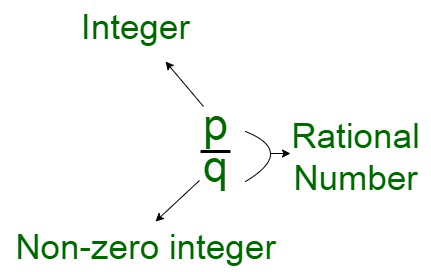# What is the density property of rational numbers?

• Last Updated : 02 Dec, 2021

Number System is a systematic way of representation of numbers of the number line. These numbers are depicted using a set of symbols and rules. The numbers lie in the interval belonging from 0-9. These numbers are also known as digits and can be manipulated using various mathematical operations. All the computations like counting, inputting, and manipulating can be performed using calculations of the number line.

Rational Numbers

A rational number is a number that is denoted in the form of a fraction, p/q where p and q are integers and also, q is not equal to 0. The entire set of rational numbers in the number system is denoted by the letter Q. In other words, In case, a number can be expressed as a fraction where both the numerator and the denominator are integral values, the number is called a rational number. These rational numbers can also be simplified further to obtain pure whole numbers or decimal values.Density Property

If a/b < c/d, then assumingly, there exists a rational number g/h such that it follows the property, a/b < g/h < c/d

The density property states that in between two specified rational numbers, there exists another rational number. For example, for given two rational numbers, 0 and 1/2 there exists a rational number 1/4 between these two rational numbers. On arranging these rational numbers in increasing order,

0, 1/4, 1/2

The rational number between the given rational numbers, 0 and 1/4 is 1/8 and the rational numbers between 1/4 and 1/2 is equivalent to 3/8.

The rational numbers between the given set of rational numbers can be computed by calculating the average of the two rational numbers.

For example, in order to compute the rational number between 0 and 1/4, we just add the rational numbers, 0 and 1/4, and then divide the sum by the integral value 2.

0 + 1/4 = 1/4

Now divide 1/4 by 2

1/4 ÷ 2 = 1/4 ÷ 2/1

1/4 ÷ 2 = 1/4 × 1/2

1/4 ÷ 2 = (1 × 1)/(4 × 2)

1/4 ÷ 2 = 1/8

In order to compute the rational number between 1/4 and 1/2, just add 1/4 and 1/2 and then divide the sum by 2, we obtain,

1/4 + 1/2 = 1/4 + (1 × 2)/(2 × 2)

1/4 + 1/2 = 1/4 + 2/4

1/4 + 1/2 = (1 + 2)/4

1/4 + 1/2 = 3/4

Now dividing 3/4 by 2,

3/4 ÷ 2 = 3/4 ÷ 2/1

3/4 ÷ 2 = 3/4 × 1/2

3/4 ÷ 2 = (3 × 1)/(4 × 2)

3/4 ÷ 2 = 3/8

### Sample Questions

Question 1. Find a rational number between 1/2 and 2/3?

Solution:

Here we have to find a rational number between 1/2 and 2/3

First take the average of both the rational numbers

= (1/2 + 2/3)/2

Taking LCM of 2 and 3

LCM of 2 and 3 = 6

= ((1×3)+(2×2)/6 )/2

= 3+4/6/2

= 7/6 × 1/2

= 7/12

Therefore,

Rational number between 1/2 and 2/3 is 7/12.

Question 2. Find two rational numbers between 3/4 and 5/8?

Solution:

Here we have to find a rational number between 3/4 and 5/8

First take the average of both the rational numbers

= (3/4 + 5/8)/2

Taking LCM of 4 and 8

LCM of 4 and 8 = 8

= ((3×2)+(5×1)/8 )/2

= 6+5/8/2

= 11/8 × 1/2

= 11/16

Now finding the other rational number between 3/4 and 11/16

First take the average of both the rational numbers

= (3/4 + 11/16)/2

Taking LCM of 4 and 16

LCM of 4 and 16 = 16

= ((3×4)+(11×1)/16 )/2

= 12+11/16/2

= 23/16 × 1/2

= 23/32

Therefore,

Rational number between 3/4 and 5/8 are 11/16 and 23/32.

Question 3. Find three rational numbers between 4/3 and 5/4?

Solution:

Here we have to find a rational number between 4/3 and 5/4

First take the average of both the rational numbers

= (4/3 + 5/4)/2

Taking LCM of 3 and 4

LCM of 3 and 4 = 12

= ((4×4)+(5×3)/12 )/2

= 16+15/12/2

= 31/12 × 1/2

= 31/24

Now finding the second rational number between 4/3 and 31/24

First take the average of both the rational numbers

= (4/3 + 31/24)/2

Taking LCM of 3 and 24

LCM of 3 and 24 = 24

= ((4×6)+(31×1)/24 )/2

= 24+31/24/2

= 55/24 × 1/2

= 55/48

Further for finding third rational number between 31/24 and 5/4

First take the average of both the rational numbers

= (31/24 + 5/4)/2

Taking LCM of 24 and 4

LCM of 24 and 4 = 24

= ((31×1)+(5×6)/24 )/2

= 31+30/24/2

= 61/24 × 1/2

= 61/48

Therefore,

Ration numbers between 4/3 and 5/4 are 55/48, 31/24, and 61/48.

My Personal Notes arrow_drop_up